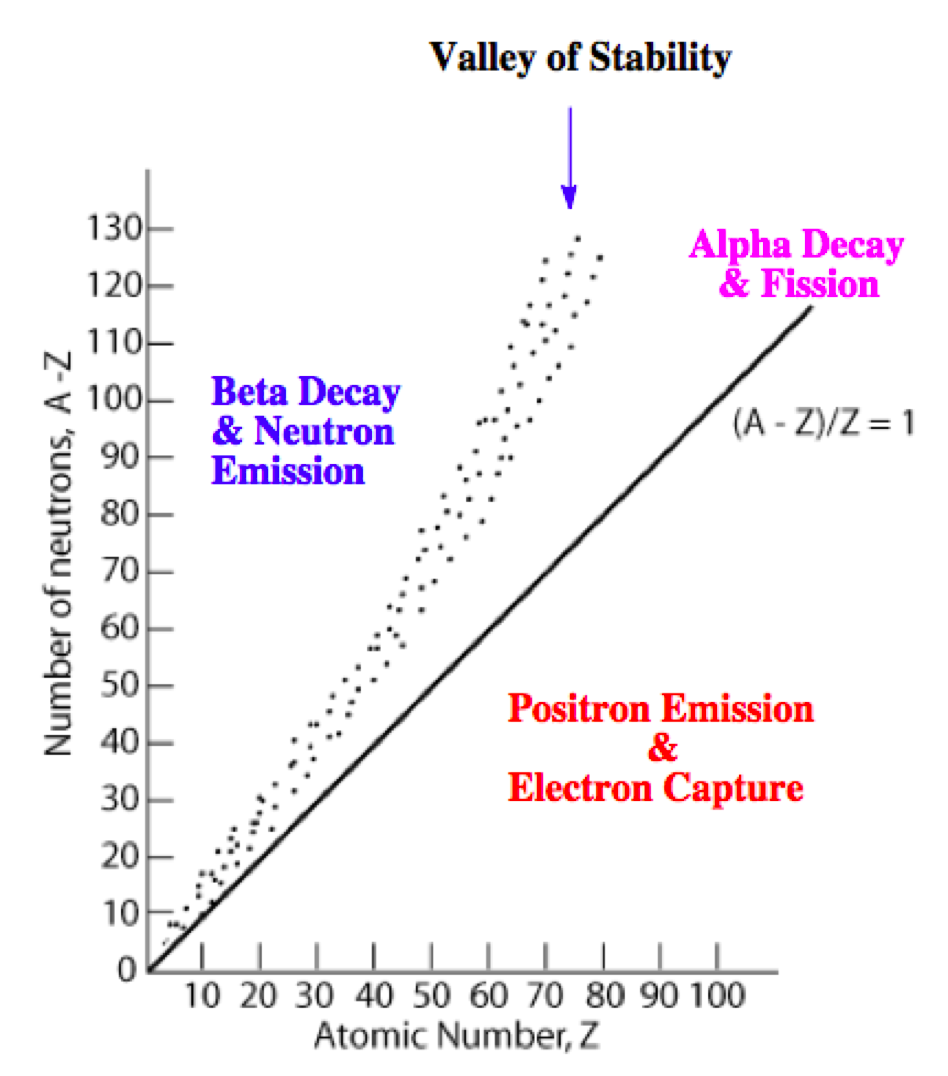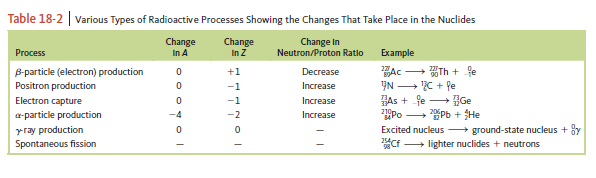# Problem: There are four stable isotopes of iron with mass numbers 54, 56, 57, and 58. There are also two radioactive isotopes: iron-53 and iron-59. Predict modes of decay for these two isotopes. (See in the following table.)

###### FREE Expert Solution

Recall that the stability of an isotope depends on the ratio of neutrons to protons (N/Z). This means for stable isotopes with:

• Z ≤ 20; the N/Z ratio should be equal to 1.0

• 20 < Z ≤ 40; the N/Z ratio should be equal to 1.25

• 40 < Z ≤ 80; the N/Z ratio should be equal to 1.50

• Z < 83; the stable nuclide does not exist

The N/Z values for stable isotopes form the valley or band of stability:This means for isotopes:

• above the valley of stability: there too many neutrons in the isotope

Mode of decay: Either beta decay (0–1β) or neutron emission (10n; not in the choices)

• below the valley of stability: there too many protons in the isotope

Mode of decay: Either positron emission (01e) or electron capture (0–1e)

• with atomic mass ≥ 200 amu: there too many protons and neutrons in the isotope

Mode of decay: alpha decay (42α)

96% (203 ratings)###### Problem Details

There are four stable isotopes of iron with mass numbers 54, 56, 57, and 58. There are also two radioactive isotopes: iron-53 and iron-59. Predict modes of decay for these two isotopes. (See in the following table.)Frequently Asked Questions

What scientific concept do you need to know in order to solve this problem?

Our tutors have indicated that to solve this problem you will need to apply the Band of Stability concept. You can view video lessons to learn Band of Stability. Or if you need more Band of Stability practice, you can also practice Band of Stability practice problems.

What professor is this problem relevant for?

Based on our data, we think this problem is relevant for Professor Scott's class at UCSC.

What textbook is this problem found in?

Our data indicates that this problem or a close variation was asked in Chemistry: An Atoms First Approach - Zumdahl Atoms 1st 2nd Edition. You can also practice Chemistry: An Atoms First Approach - Zumdahl Atoms 1st 2nd Edition practice problems.